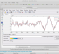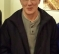## Average Impulse Response from multiple measurementsStarted by 4 years ago9 replieslatest reply 4 years ago621 views

I have taken several log sine sweep measurements in succession, long enough for the. room noise to settle to below hearing threshold before the next measurement is triggered.

I am looking at analysing the frequency and phase components of these measurements, but to do nothing more, no reconstruction.  My question is, how do I successfully average these measurements. I can take an FFT of each of them and I believe averaging the complex result of the three will give me the mean average, which I can use to extract phase and magnitude.  My question is, can I average this at an earlier stage?  Can I somehow get the average impulse response, so that I am only performing the FFT once?

[ - ]Hello Samp17. I'm sure someone can answer your question is you would tell us, in detail, EXACTLY what you are doing. You wrote that you have "taken several log sine sweep measurements in succession." What exactly does your word "taken" mean? What does your phrase "log sine sweep measurements in succession" mean?

If your processing is simply ‘averaging’ as dszabo says, then the linearity property of the DFT tells us you can average in the time-domain or average in the freq-domain. But that notion is based on the assumption that your multiple time-domain signals are synchronized in time. In that case, time-domain averaging will require fewer computations than freq-domain averaging.

[ - ]Hi

as some above have stated the linearity of the DFT tells us that averaging the measurement results in the time domain or in the frequency domain is equivalent. But this is only true if in the time domain, you superpose two measurements with exact phase. In other words - you should know the exact time delay in samples between two consecutive sweeps, then window (or cut out) the single measurement results so that the sweep starts at the same sample for all your measurements. Then you can just add them up and devide by the number of measurements.

[ - ]Hi dudelsound. You are correct. And your words "the sweep starts at the same sample for all your measurements" are equivalent to my words "multiple time-domain signals are synchronized in time."

[ - ]As stated, averaging is a type of filtering, so averaging in the time domain is equivalent to multiplication in the frequency domain. However, given that you are averaging multiple time domain responses, I would view the problem a bit differently.  Basically, you are taking multiple impulse responses and summing them, then scaling them by dividing by the number of impulse responses.  Both scaling and summing are equivalent in the time and frequency domains.  Based on that, you should get the same result if you do it in the time or frequency domains.  You should be able to check that pretty easily.  This only applies if you are working with the complex data in the frequency domain, not the phase/magnitude independently.  Best of luck

[ - ]Hi,

If I were to do that, I would first check in time domain the similarities-differences of the impulse responses. Then in frequency domain, I would check differences for the impulse responses. Assuring after a 'certain degree' of similarity, averaging could be applied?

[ - ]Samp17-

You can think of averaging in the time domain as somewhat of a convolution.  For thought purposes, consider one of your impulses as the input and another as the filter.  Convolution in the time domain is multiplication in the frequency domain, so that would not be what you want.  It would be fine if one of your impulses is a filter of some type, but that's not the case.

-Jeff

[ - ]Hi Jeff,

that makes sense to me. But still leaves me confused as to how to average measurements.  Most software out there do averaging in some form.  So you will select how many sweeps to take, and the software will average out the results. I can take the individual sweeps, but do not know how to average.  Unless just averaging the complex numbers of the individual results. But that will obviously take a lot more computational time if I manipulate the data in some way, I will have to FFT and then calculate the average for every change.

If that's the correct way to do this, then I shall continue down that avenue, but wanted to check if I could somehow average out the impulse early on.

[ - ]Hello,

You can determine the autocorrelation of the excitation and the cross correlation input / output for each data block. You can then average these and improve the SNR. Then calculate the ratio of the FFT of the cross-correlation and the FFT of the autocorrelation to obtain the transfer function of the system. An inverse FFT then gives the impulse response.

Regards

[ - ]An example of such identification:

% Skript identif_2.m,

clear;

% ------ Signals

N = 1024;

%x = sign(randn(1,N));

x = randn(1,N);

% System

nord = 64;

h = fir1(nord, 0.2);  % LP-Filter (Lowpass)

y = filter(h,1,x);

figure(1);    clf;

subplot(211), plot(0:N-1, x,'k-','LineWidth',1);

title('Inputsignal');  grid on;

subplot(212), plot(0:N-1, y,'k-','LineWidth',1);

title('Outputsignal');  grid on;

% ------ Correlationfunction

Rxx = xcorr(x,x,nord);   % Autokorrelation

nxx = length(Rxx);

Rxy = xcorr(x,y,nord);   % Crosscorrelation

nxy = length(Rxy);

figure(2);    clf;

subplot(211), plot(0:nxx-1, Rxx,'k-','LineWidth',1);

title('Autocorrelation');  grid on;

subplot(212), plot(0:nxy-1, Rxy,'k-','LineWidth',1);

title('Crosscorrelation');  grid on;

% ------ Transferfunction

Hxx = fft(Rxx);      Hxy = fft(Rxy);

Hs = Hxy./Hxx;

hg = real(ifft(Hs));

figure(3);    clf;

subplot(211), plot(0:nord, h,'k-','LineWidth',1);

title('Impuls response of the Systems');   grid on;

subplot(212), plot(0:nxx-1, fftshift(hg),'k-','LineWidth',1);

title('Estimated impuls response of the Systems');   grid on;